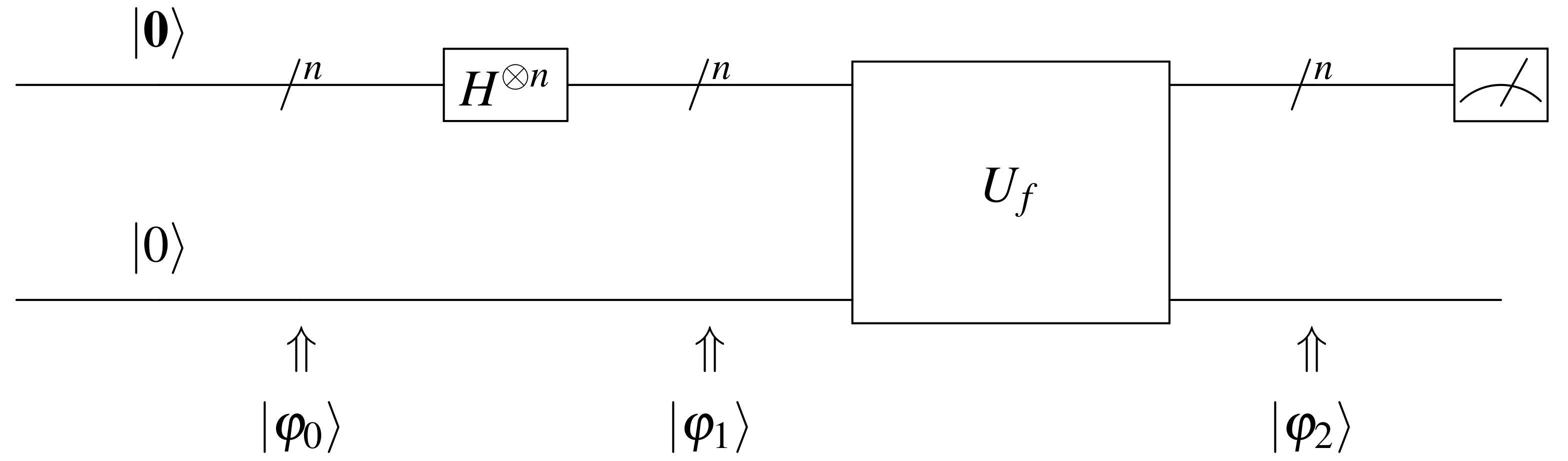# Drawing Quantum Circuit Using Q-Circuit

## Introduction

Q-circuit is a macro package for drawing quantum circuit diagrams in $\rm\LaTeX$. In this blog post, I would present a simple example of quantum circuit drawn using Q-circuit.

## Example

In this particular example, there is a quantum oracle $U_f$ in the circuit. We wrote the following $\rm\LaTeX$ code for drawing the quantum circuits. It should be noted that mathptmx makes the math symbol font Times and it looks much better.

The quantum circuit diagram created is as follows.## Resources

More examples and resources could be found in the Q-circuit tutorial in the references.